# Calling table functions from Microsoft Excel

This article explains how the table functions of the non-commercial .net library finaquant® protos can be called from within Microsoft Excel using VBA macros.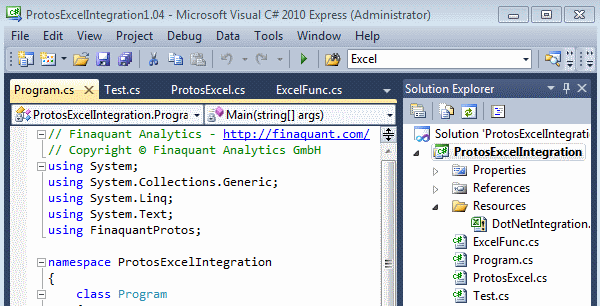Two possible integration options are presented here:

1) Calling a .net method from an Excel macro
Once called from a macro, the .net method reads the input tables from the given excel worksheets before executing the table functions to obtain output tables. The output tables are in turn written into the desired excel sheets.

Note that names of the worksheets are passed from the macro to the .net method as parameters for selecting the input tables. Each worksheet contains a single input table.

This integration option can be useful for demonstrations that are executed directly from excel. See the code examples below:

Excel VBA macro calling .net method: CalculatePrices()
```' Calculate price table with given cost and margin tables ' 1) Read input tables: Costs, Margins, MetaData ' 2) Calculate price table ' 3) Write resultant price table into a sheet Private Sub CalculatePrices() Dim pxl As New ProtosExcelFunc Dim CostTableSheet, MarginTableSheet, MetaDataSheet, PriceTableSheet As String   CostTableSheet = "CostTable" MetaDataSheet = "MetaData"   MarginTableSheet = "MarginTable1" PriceTableSheet = "PriceTable1"   'MarginTableSheet = "MarginTable2" 'PriceTableSheet = "PriceTable2"   ' call .net method MsgBox pxl.CalculatePrices(Application.ActiveWorkbook.FullName, CostTableSheet, _ MarginTableSheet, MetaDataSheet, PriceTableSheet) End Sub```
.net method called by Excel macro: CalculatePrices()
```/// <summary> /// Calculate price table for given cost and margin tables /// </summary> /// <param name="ExcelFilePath">Full file path of excel file</param> /// <param name="CostTableSheet">Name of worksheet for reading cost table (input table)</param> /// <param name="MarginTableSheet">Name of worksheet for reading margin table (input table)</param> /// <param name="MetaDataSheet">Name of worksheet for reading field names and types (input table)</param> /// <param name="PriceTableSheet">Name of target worksheet for writing price table (output table)</param> /// <returns>Message to calling function</returns> public string CalculatePrices(string ExcelFilePath, string CostTableSheet, string MarginTableSheet, string MetaDataSheet, string PriceTableSheet) { try { // read meta data DataTable MetaTbl = ExcelFunc.ReadTableFromExcelSheet_ODBC(ExcelFilePath, MetaDataSheet); MetaData md = MetaData.ReadFieldsFromDataTable(MetaTbl);   // read input tables DataTable CostTbl = ExcelFunc.ReadTableFromExcelSheet_ODBC(ExcelFilePath, CostTableSheet); DataTable MarginTbl = ExcelFunc.ReadTableFromExcelSheet_ODBC(ExcelFilePath, MarginTableSheet);   // convert DataTable to MatrixTable MatrixTable CostTable = MatrixTable.Import_from_DataTable(CostTbl, md); MatrixTable MarginTable = MatrixTable.Import_from_DataTable(MarginTbl, md);   // Calculate price table with table function 'MultiplySelectedKeyFigures' MatrixTable PriceTable = MatrixTable.MultiplySelectedKeyFigures(CostTable, (MarginTable + 1), InputKeyFigTbl1: "costs", InputKeyFigTbl2: "margin", OutputKeyFig: "price", JokerMatchesAllvalues: true);   // convert MatrixTable to DataTable DataTable PriceTbl = MatrixTable.Export_To_DataTable(PriceTable);   // write price table into excel sheet ExcelFunc.WriteTableToExcelSheet(PriceTbl, ExcelFilePath, SheetName: PriceTableSheet, SaveWorkbook: false);   // return affirmative message to caller return "Price table is calculated successfully!"; } catch (Exception ex) { return "CalculatePrices: An error has occured! " + ex.Message; } }```

2) Directly executing the .net method
… which reads input tables from the given excel file before making the required table calculations.

In this option, an excel sheets are used solely as a database to store input and output tables; no additional VBA code (Excel macro) is needed. See the code example below:

.net code for calculating prices: CalculatePriceTable()
```/// <summary> /// Calculate price table with given cost and margin tables /// </summary> public static void CalculatePriceTable() { // Get full file path string filepath = System.IO.Path.GetFullPath(@"Resources\DotNetIntegration.xlsm");   // call table function ProtosExcelFunc pxl = new ProtosExcelFunc(); string msg = pxl.CalculatePrices(filepath, CostTableSheet: "CostTable", MarginTableSheet: "MarginTable1", MetaDataSheet: "MetaData", PriceTableSheet: "PriceTable1");   System.Diagnostics.Debug.WriteLine("Message from CalculatePrices: " + msg); }```

All the example codes above and others can be found in MS Visual Studio File ProtosExcelIntegration (1570 downloads) .

### Calling a .net method from an Excel macro

1. Install the latest release of the non-commercial .net library finaquant® protos on your computer.
2. Ensure that the proper ODBC driver is installed on your computer. See related article for more information.
4. Open the VS file ProtosExcelIntegration as administrator: Find the application (Visual Studio) in program starter menu, right mouse-click and select Run as administrator.
5.6. Ensure that Microsoft Office and Excel Object libraries are referenced by the VS project. You need to update references if you have a Microsoft Office version other than 2010.
7.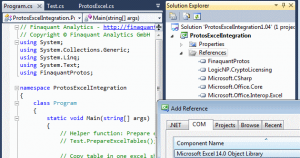8. Ensure that following VS project configurations are selected:
9. Application>Output type: Class Library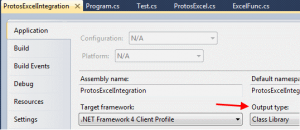Build>Output: Check Register for COM interop10. Build (or rebuild) the VS project.
11.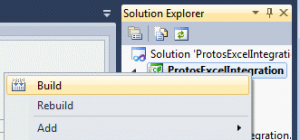12. Add library COM reference to Excel: Open the excel file under the folder Resources, go to macro VBA view, add reference named Finaquant Protos and Excel Integration from the menu Tools>References
13.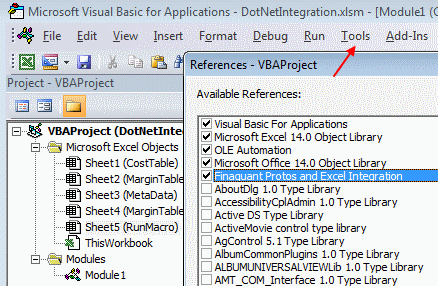You can now open the excel file under the folder Resources and execute some macros (by pressing F5) that call .net methods for table functions. The VBA macros can be found in Module1 in developer view: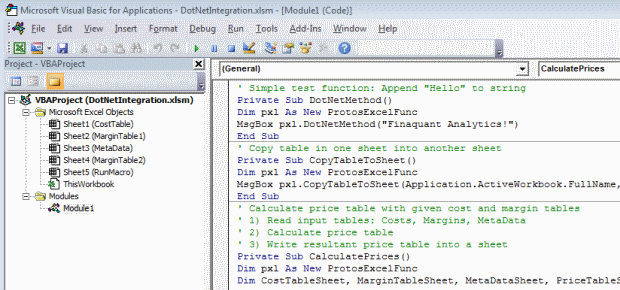You can also execute a macro (CalculatePrices2 in Module1) by selecting the input tables (by their worksheet names) and pressing a button in the worksheet named RunMacro: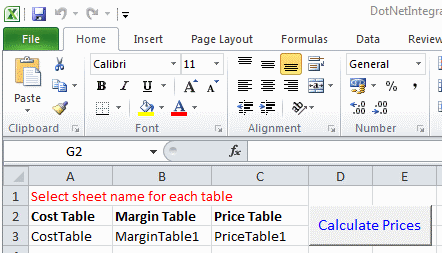Resultant price table is written into a new worksheet named ProceTable1: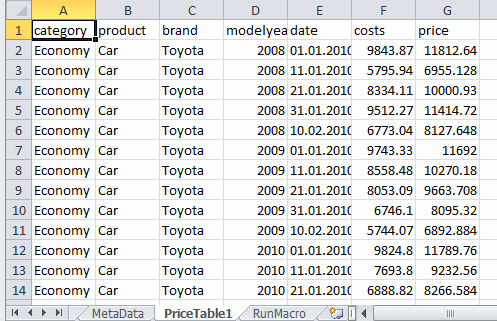This integration option is particularly useful for demonstrations: Users can first enter the calculation parameters (like sheet names for selecting the input tables here) and execute their table calculation by just pressing a button.

### Directly executing the .net method

1. Open the VS file ProtosExcelIntegration as normal user; you don’t need to open it as administrator.
2. Ensure that following VS project configurations are selected:
Build>Output: Uncheck Register for COM interop
Application>Output type: Select Console Application
3. Uncomment the test method you want to execute in code file Program.cs and press F5
4.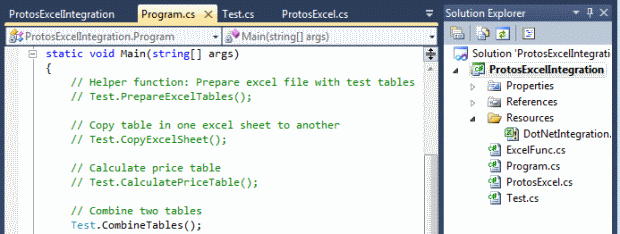Resultant table is written into a new worksheet named CombTable2: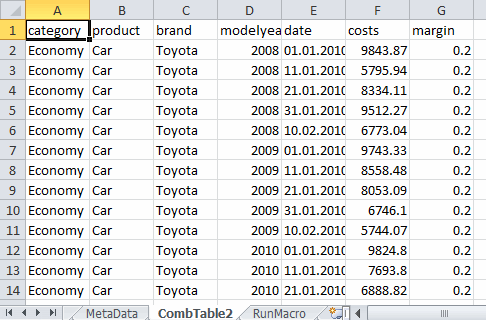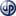Copyright secured by Digiprove © 2013 Tunc Ali Kütükcüoglu
This entry was posted in Calculation engine and tagged , , , . Bookmark the permalink.

### 1 Response to Calling table functions from Microsoft Excel

1.vargas says:

Calculation engine with multiple calculation instances is designed to work with a relational database, right? Can it also work with an excel file?